# Clear handwriting The Zn-S-O-H20 Eh-pH diagram and the hydroxide precipitation diagram shown below from the literature...

Clear handwriting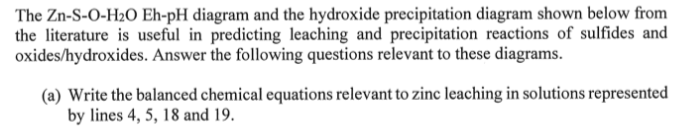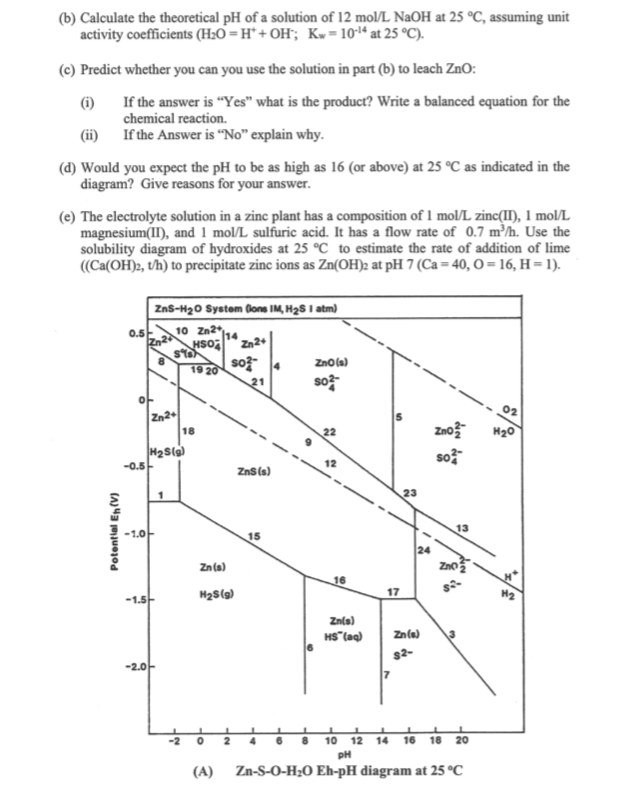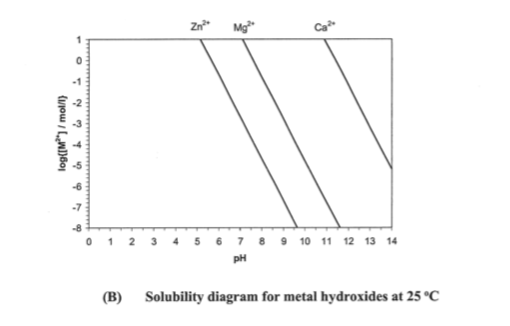The Zn-S-O-H20 Eh-pH diagram and the hydroxide precipitation diagram shown below from the literature is useful in predicting leaching and precipitation reactions of sulfides and oxides/hydroxides. Answer the following questions relevant to these diagrams (a) Write the balanced chemical equations relevant to zinc leaching in solutions represented by lines 4, 5, 18 and 19
(b) Calculate the theoretical pH of a solution of 12 mol/L NaOH at 25 °C, assuming unit activity coefficients (H2O=H*+OH;; K = 1014 at 25 °C). (c) Predict whether you can you use the solution in part (b) to leach ZnO: If the answer is "Yes" what is the product? Write a balanced equation for the chemical reaction. (i) If the Answer is "No" explain why. (ii) (d) Would you expect the pH to be as high as 16 (or above) at 25 °C as indicated in the diagram? Give reasons for your answer. (e) The electrolyte solution in a zinc plant has a composition of 1 mol/L zinc(II), 1 mol/L magnesium(II), and 1 mol/L sulfuric acid. It has a flow rate of 0.7 m/h. Use the solubility diagram of hydroxides at 25 C to estimate the rate of addition of lime ((Ca(OH)2, th) to precipitate zinc ions as Zn(OH)2 at pH 7 (Ca 40, O0= 16, H= 1) ZnS-H20 System Gons IM, H2S I atm) 0.5 10 Zn2 2 NSO n2 ZnO(a) 19 20 so Zn2 18 Zno H20 22 H2S(9) -0.5 so 12 ZnS(s) 1 13 15 24 Zno Zn(s) 16 17 H2S() H2 Znle) HS (aq) Zn(a) s2- -2.0 10 12 14 16 16 20 Zn-S-O-H2o Eh-pH diagram at 25 °C (A) Potential En(V)
Zn2 Mg 10 11 12 13 14 pH (B) Solubility diagram for metal hydroxides at 25 °C uou, Lwl)60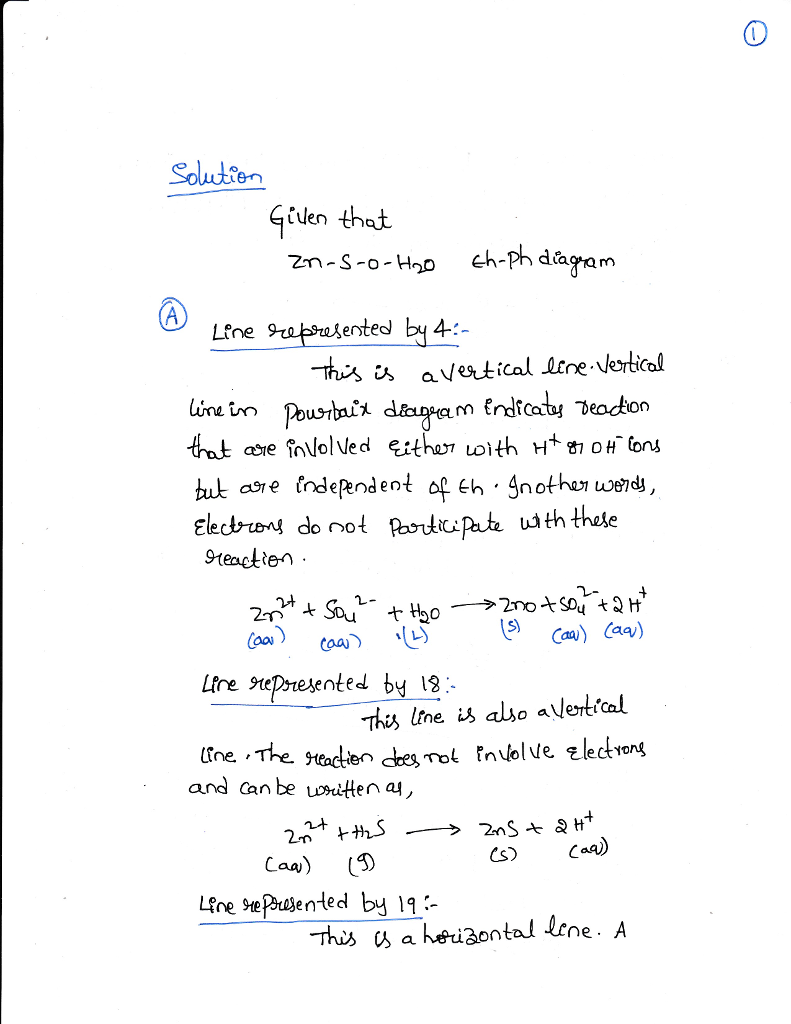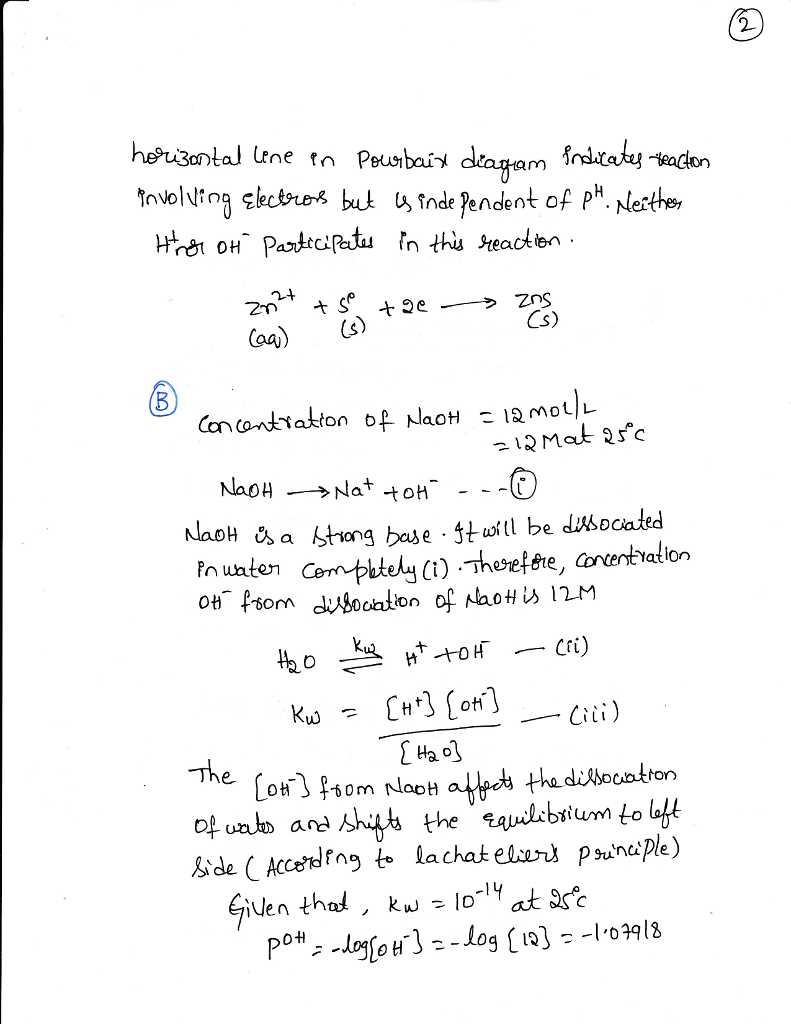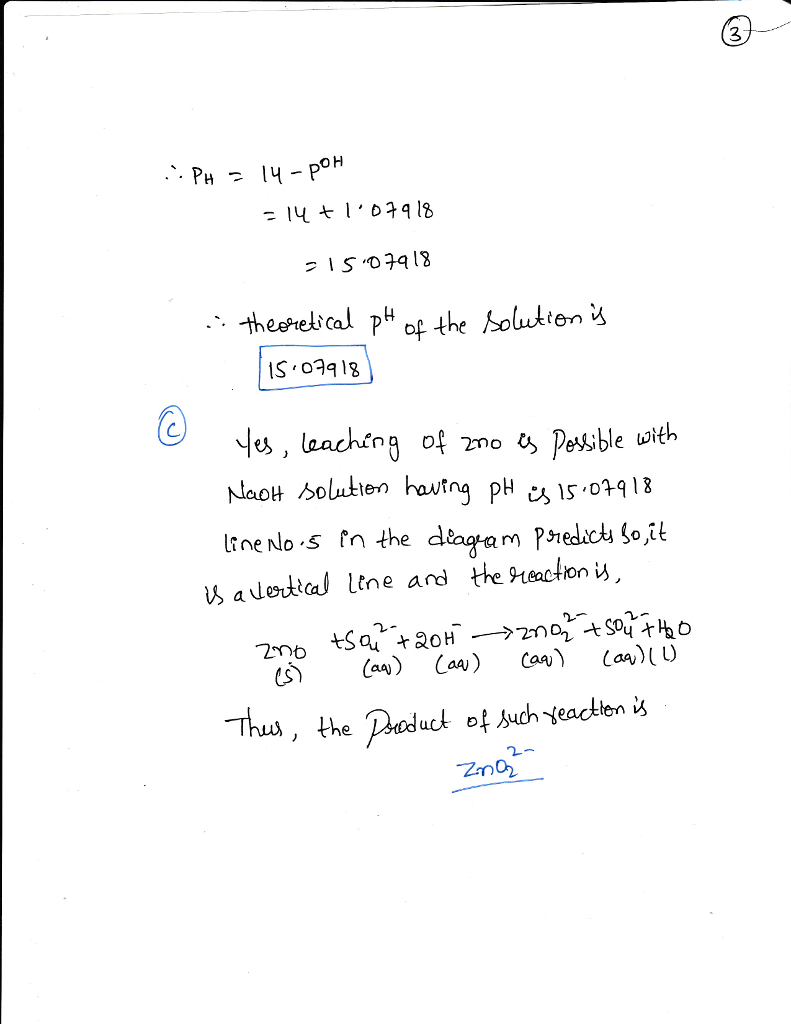##### Add Answer of: Clear handwriting The Zn-S-O-H20 Eh-pH diagram and the hydroxide precipitation diagram shown below from the literature...
Similar Homework Help Questions
• ### 3.2 Reactions of precipitates of Ag(l), Ni(lI), Zn(lI) with acid/base (a) Label two test tubes with Ag(l). Repeat with Ni(Il), and Zn(). Place 6-7 drops of the following metal ion solutions into the...3.2 Reactions of precipitates of Ag(l), Ni(lI), Zn(lI) with acid/base (a) Label two test tubes with Ag(l). Repeat with Ni(Il), and Zn(). Place 6-7 drops of the following metal ion solutions into the appropriate test tubes. (i)silver nitrate solution (ü) zinc chloride solution (ii) nickel(lI) sulfate solution (b) Add to each of the six solutions 2 mol L-1 aqueous NaOH one drop at a time until a precipitate is formed. Add 2 mol L-1 aqueous HNOs dropwise to one test...

• ### References A solution is 0.0150M in Pb ions. If 0.140 mol of solid Na2 SO4 is...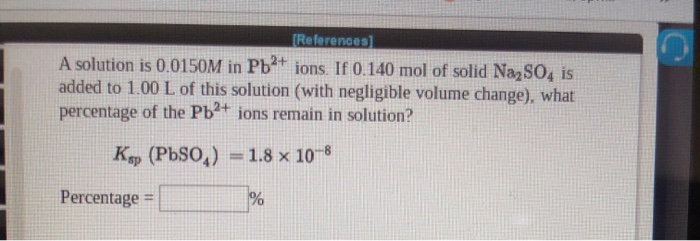References A solution is 0.0150M in Pb ions. If 0.140 mol of solid Na2 SO4 is added to 1.00 L of this solution (with negligible volume change), what percentage of the Pbt ions remain in solution? Kp (PbSO,) 1.8 x 10-8 Percentage = Solubility Product Constants for Some Inorganic Compounds at 25°C APPENDIX Substance Substance Aluminum compounds AIAsO AlOH) к, Chromium compeands CrAsO, CrOH) CrPO Cabalt compounds Co(AsOd CoCO, Co(OH) CoS (a) CoS () Co(OH), 1.6 x 10 1 1.9...

• ### let me know if you need any values Review Topics References) Use the References to access important values if...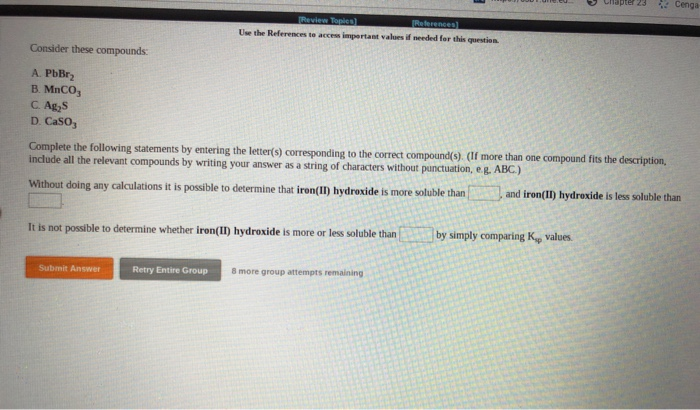let me know if you need any values Review Topics References) Use the References to access important values if needed for this question Consider these compounds A. PbBr2 B. MnCO; C. Ag, D. Caso, Complete the following statements by entering the letter(s) corresponding to the correct compound(s). (If more than one compound fits the description, include all the relevant compounds by writing your answer as a string of characters without punctuation, e.. ABC) Without doing any calculations it is possible...

• ### 4.30. Using solubility rules, predict the solubility in water of the following ionic compounds. a. AI(OH) b. CaN...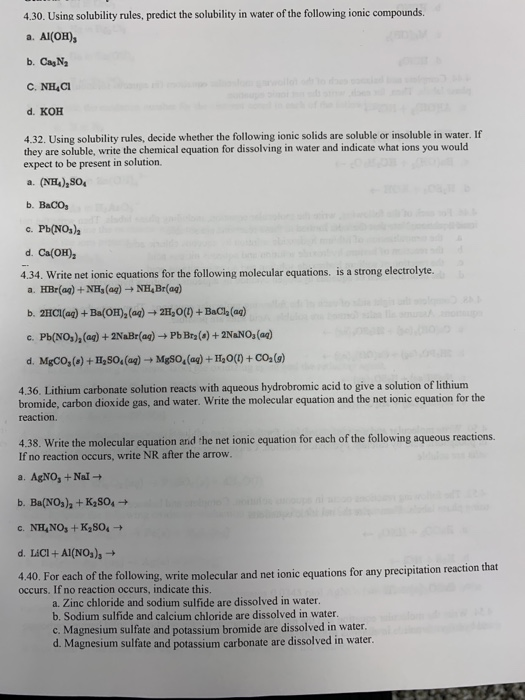4.30. Using solubility rules, predict the solubility in water of the following ionic compounds. a. AI(OH) b. CaN C. NH4CI d. KOH 4.32. Using solubility rules, decide whether the following ionic solids are soluble or insoluble in water. If they are soluble, write the chemical equation for dissolving in water and indicate what ions you would expect to be present in solution. (NE SO b. BaCO c. Pb(NOs)2 d. Ca(OH) 4.34. Write net ionic equations for the following molecular equations....

• ### What is the coefficient of H.So, when the following equation is properly balanced with the smallest set of w...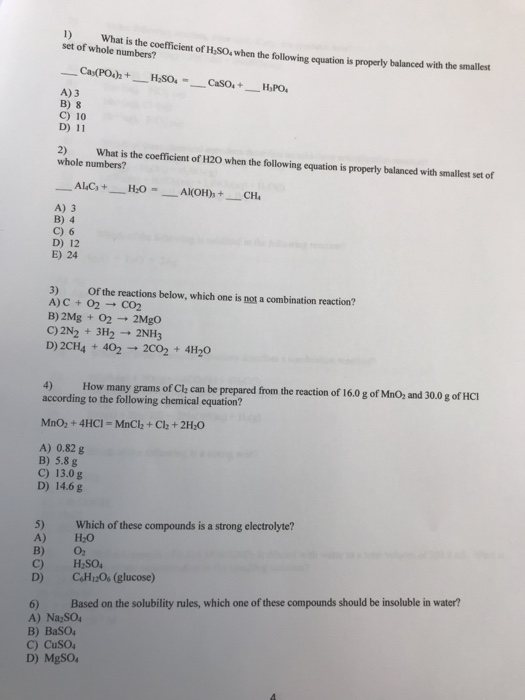What is the coefficient of H.So, when the following equation is properly balanced with the smallest set of whole numbers? __Cas(PO4h + H2SO4 - Caso + HPO. A) 3 B) 8 C) 10 D) 11 2) What is the coefficient of H20 when the following equation is properly balanced with smallest set of whole numbers? ALC + H2O - AM(OH), + CHE A) 3 B) 4 C) 6 D) 12 E) 24 3) of the reactions below, which one is...

Need Online Homework Help?